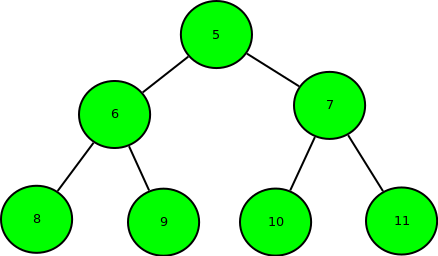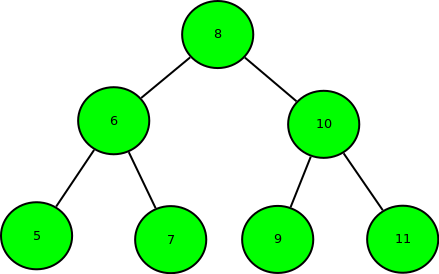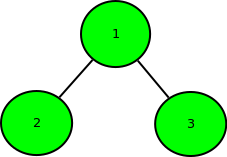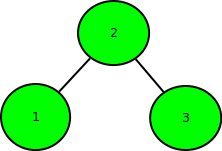Related Articles

# Minimum swap required to convert binary tree to binary search tree

• Difficulty Level : Hard
• Last Updated : 11 Jul, 2021

Given the array representation of Complete Binary Tree i.e, if index i is the parent, index 2*i + 1 is the left child and index 2*i + 2 is the right child. The task is to find the minimum number of swap required to convert it into Binary Search Tree.

Examples:

```Input : arr[] = { 5, 6, 7, 8, 9, 10, 11 }
Output : 3
Binary tree of the given array:``````Swap 1: Swap node 8 with node 5.
Swap 2: Swap node 9 with node 10.
Swap 3: Swap node 10 with node 7.``````So, minimum 3 swaps are required.

Input : arr[] = { 1, 2, 3 }
Output : 1
Binary tree of the given array:````After swapping node 1 with node 2.``So, only 1 swap required.`

The idea is to use the fact that inorder traversal of Binary Search Tree is in increasing order of their value.
So, find the inorder traversal of the Binary Tree and store it in the array and try to sort the array. The minimum number of swap required to get the array sorted will be the answer. Please refer below post to find minimum number of swaps required to get the array sorted.
Minimum number of swaps required to sort an array
Time Complexity: O(n log n).

## C++

 `// C++ program for Minimum swap required``// to convert binary tree to binary search tree``#include``using` `namespace` `std;` `// Inorder Traversal of Binary Tree``void` `inorder(``int` `a[], std::vector<``int``> &v,``                        ``int` `n, ``int` `index)``{``    ``// if index is greater or equal to vector size``    ``if``(index >= n)``        ``return``;``    ``inorder(a, v, n, 2 * index + 1);``    ` `    ``// push elements in vector``    ``v.push_back(a[index]);``    ``inorder(a, v, n, 2 * index + 2);``}` `// Function to find minimum swaps to sort an array``int` `minSwaps(std::vector<``int``> &v)``{``    ``std::vector > t(v.size());``    ``int` `ans = 0;``    ``for``(``int` `i = 0; i < v.size(); i++)``        ``t[i].first = v[i], t[i].second = i;``    ` `    ``sort(t.begin(), t.end());``    ``for``(``int` `i = 0; i < t.size(); i++)``    ``{``        ``// second element is equal to i``        ``if``(i == t[i].second)``            ``continue``;``        ``else``        ``{``            ``// swaping of elements``            ``swap(t[i].first, t[t[i].second].first);``            ``swap(t[i].second, t[t[i].second].second);``        ``}``        ` `        ``// Second is not equal to i``        ``if``(i != t[i].second)``            ``--i;``        ``ans++;``    ``}``    ``return` `ans;``}` `// Driver code``int` `main()``{``    ``int` `a[] = { 5, 6, 7, 8, 9, 10, 11 };``    ``int` `n = ``sizeof``(a) / ``sizeof``(a);``    ``std::vector<``int``> v;``    ``inorder(a, v, n, 0);``    ``cout << minSwaps(v) << endl;``}` `// This code is contributed by code_freak`

## Python3

 `# Python3 program for Minimum swap required``# to convert binary tree to binary search tree` `# Inorder Traversal of Binary Tree``def` `inorder(a, n, index):``    ` `    ``global` `v``    ` `    ``# If index is greater or equal to``    ``# vector size``    ``if` `(index >``=` `n):``        ``return``    ` `    ``inorder(a, n, ``2` `*` `index ``+` `1``)` `    ``# Push elements in vector``    ``v.append(a[index])``    ``inorder(a, n, ``2` `*` `index ``+` `2``)` `# Function to find minimum swaps``# to sort an array``def` `minSwaps():``    ` `    ``global` `v``    ``t ``=` `[[``0``, ``0``] ``for` `i ``in` `range``(``len``(v))]``    ``ans ``=` `-``2` `    ``for` `i ``in` `range``(``len``(v)):``        ``t[i][``0``], t[i][``1``] ``=` `v[i], i` `    ``t, i ``=` `sorted``(t), ``0` `    ``while` `i < ``len``(t):``        ` `        ``# break``        ``# second element is equal to i``        ``if` `(i ``=``=` `t[i][``1``]):``            ``i ``+``=` `1``            ``continue``        ``else``:``            ` `            ``# Swaping of elements``            ``t[i][``0``], t[t[i][``1``]][``0``] ``=` `t[t[i][``1``]][``0``], t[i][``0``]``            ``t[i][``1``], t[t[i][``1``]][``1``] ``=` `t[t[i][``1``]][``1``], t[i][``1``]` `        ``# Second is not equal to i``        ``if` `(i ``=``=` `t[i][``1``]):``            ``i ``-``=` `1` `        ``i ``+``=` `1` `        ``ans ``+``=` `1` `    ``return` `ans` `# Driver Code``if` `__name__ ``=``=` `'__main__'``:``    ` `    ``v ``=` `[]``    ``a ``=` `[ ``5``, ``6``, ``7``, ``8``, ``9``, ``10``, ``11` `]``    ``n ``=` `len``(a)``    ``inorder(a, n, ``0``)` `    ``print``(minSwaps())` `# This code is contributed by mohit kumar 29`

## Javascript

 ``

## Java

 `// Java program for Minimum swap required``// to convert binary tree to binary search tree``import` `java.util.*;` `public` `class` `GFG{` `    ``// Pair class``    ``static` `class` `Pair{``        ``int` `first, second;` `        ``Pair(``int` `a, ``int` `b){``            ``first = a;``            ``second = b;``        ``}``    ``}``    ` `    ``// Inorder Traversal of Binary Tree``    ``static` `void` `inorder(``int` `a[], Vector v, ``int` `n, ``int` `index)``    ``{``        ``// if index is greater or equal to vector size``        ``if``(index >= n)``            ``return``;``            ` `        ``inorder(a, v, n, ``2` `* index + ``1``);``        ` `        ``// push elements in vector``        ``v.add(a[index]);``        ` `        ``inorder(a, v, n, ``2` `* index + ``2``);``    ``}``    ` `    ``// Function returns the``    ``// minimum number of swaps``    ``// required to sort the array``    ``// Refer :``    ``// https://www.geeksforgeeks.org/minimum-number-swaps-required-sort-array/``    ``public` `static` `int` `minSwaps(Vector arr)``    ``{``        ``int` `n = arr.size();`` ` `        ``ArrayList < Pair > arrpos = ``new` `ArrayList < Pair > ();``        ``for` `(``int` `i = ``0``; i < n; i++)``             ``arrpos.add(``new` `Pair(arr.get(i), i));`` ` `        ``// Sort the array by array element values to``        ``// get right position of every element as the``        ``// elements of second array.``        ``arrpos.sort(``new` `Comparator()``        ``{``            ``@Override``            ``public` `int` `compare(Pair o1, Pair o2)``            ``{``                ``return` `o1.first - o2.first;``            ``}``        ``});`` ` `        ``// To keep track of visited elements. Initialize``        ``// all elements as not visited or false.``        ``Boolean[] vis = ``new` `Boolean[n];``        ``Arrays.fill(vis, ``false``);`` ` `        ``// Initialize result``        ``int` `ans = ``0``;`` ` `        ``// Traverse array elements``        ``for` `(``int` `i = ``0``; i < n; i++)``        ``{``            ``// already swapped and corrected or``            ``// already present at correct pos``            ``if` `(vis[i] || arrpos.get(i).first == i)``                ``continue``;`` ` `            ``// find out the number of  node in``            ``// this cycle and add in ans``            ``int` `cycle_size = ``0``;``            ``int` `j = i;``            ``while` `(!vis[j])``            ``{``                ``vis[j] = ``true``;`` ` `                ``// move to next node``                ``j = arrpos.get(j).second;``                ``cycle_size++;``            ``}`` ` `            ``// Update answer by adding current cycle.``            ``if``(cycle_size > ``0``)``            ``{``                ``ans += (cycle_size - ``1``);``            ``}``        ``}`` ` `        ``// Return result``        ``return` `ans;``    ``}` `    ``// Driver code``    ``public` `static` `void` `main(String args[])``    ``{``        ``int` `a[] = { ``5``, ``6``, ``7``, ``8``, ``9``, ``10``, ``11` `};``        ``int` `n = a.length;``        ` `        ``Vector v = ``new` `Vector();` `        ``inorder(a, v, n, ``0``);` `        ``System.out.println(minSwaps(v));``    ``}``}`
Output
```3
```

Exercise: Can we extend this to normal binary tree, i.e., a binary tree represented using left and right pointers, and not necessarily complete?

This article is contributed by Anuj Chauhan. If you like GeeksforGeeks and would like to contribute, you can also write an article using write.geeksforgeeks.org or mail your article to contribute@geeksforgeeks.org. See your article appearing on the GeeksforGeeks main page and help other Geeks.